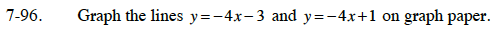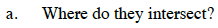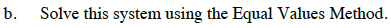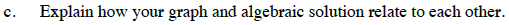### Home > CC3 > Chapter 7 > Lesson 7.3.1 > Problem7-96

7-96.
1. Graph the lines y = −4x − 3 and y = −4x + 1 on graph paper. 7-96 HW eTool (Desmos). Homework Help ✎

1. Where do they intersect?

2. Solve this system using the Equal Values Method.

3. Explain how your graph and algebraic solution relate to each other.At what coordinate do the lines cross?Set both equations equal to each other.

Get all the x terms on one side.

There is no solution. These lines do not intersect.What does your algebraic solution represent on your graph?

Use the eTool below to graph the lines.
Click the link at right for the full version of the eTool: CC3 7-96 HW eTool